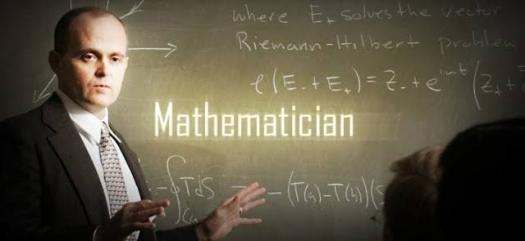# IB Mathematics Sl Prep

10 Questions | Total Attempts: 116SettingsThe IB Mathematics SL Prep is prepared to nourish your interest in mathematics. It is prepared to improve your competence. The prep contains questions related to the requirements of future exams. Want to study mathematics as a major in the university or not? This prep gets you ready.

Related Topics
• 1.
What is the sum of angles in a tight angle?
• A.

90°

• B.

120°

• C.

150°

• D.

180°

• 2.
What is the sum of angles on a straight line?
• A.

45°

• B.

270°

• C.

90°

• D.

180°

• 3.
Which of these is not a perfect square?
• A.

1225

• B.

343

• C.

2401

• D.

625

• 4.
What is the reciprocal of 2?
• A.

1

• B.

0.2

• C.

0.5

• D.

0.1

• 5.
What is the total angle at a point?
• A.

90°

• B.

180°

• C.

270°

• D.

360°

• 6.
What does the term "sum" denote in mathematical sciences?
• A.

Division

• B.

• C.

Multiplication

• D.

Subtraction

• 7.
What is the value of 'X' in this equation: 3X-3=0
• A.

0

• B.

1

• C.

2

• D.

3

• 8.
Which of these angles is complimentary of 78°?
• A.

20°

• B.

• C.

21°

• D.

12°

• 9.
What is the mode of this dataset: 2,7,9,2,10,12
• A.

2

• B.

7

• C.

9

• D.

12

• 10.
Which of these is supplementary 78°?
• A.

19°

• B.

102°

• C.

56°

• D.

23°